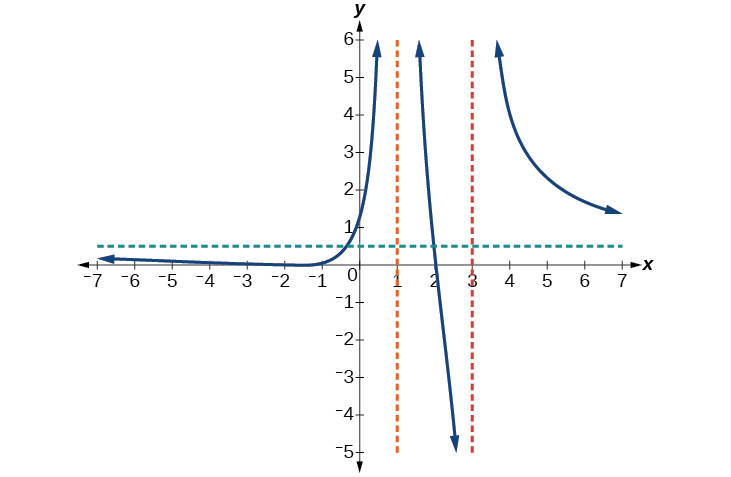# 3.7 Rational functions  (Page 8/16)

 Page 8 / 16

Given a rational function, sketch a graph.

1. Evaluate the function at 0 to find the y -intercept.
2. Factor the numerator and denominator.
3. For factors in the numerator not common to the denominator, determine where each factor of the numerator is zero to find the x -intercepts.
4. Find the multiplicities of the x -intercepts to determine the behavior of the graph at those points.
5. For factors in the denominator, note the multiplicities of the zeros to determine the local behavior. For those factors not common to the numerator, find the vertical asymptotes by setting those factors equal to zero and then solve.
6. For factors in the denominator common to factors in the numerator, find the removable discontinuities by setting those factors equal to 0 and then solve.
7. Compare the degrees of the numerator and the denominator to determine the horizontal or slant asymptotes.
8. Sketch the graph.

## Graphing a rational function

Sketch a graph of $\text{\hspace{0.17em}}f\left(x\right)=\frac{\left(x+2\right)\left(x-3\right)}{{\left(x+1\right)}^{2}\left(x-2\right)}.$

We can start by noting that the function is already factored, saving us a step.

Next, we will find the intercepts. Evaluating the function at zero gives the y -intercept:

To find the x -intercepts, we determine when the numerator of the function is zero. Setting each factor equal to zero, we find x -intercepts at $\text{\hspace{0.17em}}x=–2\text{\hspace{0.17em}}$ and $\text{\hspace{0.17em}}x=3.\text{\hspace{0.17em}}$ At each, the behavior will be linear (multiplicity 1), with the graph passing through the intercept.

We have a y -intercept at $\text{\hspace{0.17em}}\left(0,3\right)\text{\hspace{0.17em}}$ and x -intercepts at $\text{\hspace{0.17em}}\left(–2,0\right)\text{\hspace{0.17em}}$ and $\text{\hspace{0.17em}}\left(3,0\right).$

To find the vertical asymptotes, we determine when the denominator is equal to zero. This occurs when $\text{\hspace{0.17em}}x+1=0\text{\hspace{0.17em}}$ and when $\text{\hspace{0.17em}}x–2=0,\text{\hspace{0.17em}}$ giving us vertical asymptotes at $\text{\hspace{0.17em}}x=–1\text{\hspace{0.17em}}$ and $\text{\hspace{0.17em}}x=2.$

There are no common factors in the numerator and denominator. This means there are no removable discontinuities.

Finally, the degree of denominator is larger than the degree of the numerator, telling us this graph has a horizontal asymptote at $\text{\hspace{0.17em}}y=0.$

To sketch the graph, we might start by plotting the three intercepts. Since the graph has no x -intercepts between the vertical asymptotes, and the y -intercept is positive, we know the function must remain positive between the asymptotes, letting us fill in the middle portion of the graph as shown in [link] .

The factor associated with the vertical asymptote at $\text{\hspace{0.17em}}x=-1\text{\hspace{0.17em}}$ was squared, so we know the behavior will be the same on both sides of the asymptote. The graph heads toward positive infinity as the inputs approach the asymptote on the right, so the graph will head toward positive infinity on the left as well.

For the vertical asymptote at $\text{\hspace{0.17em}}x=2,\text{\hspace{0.17em}}$ the factor was not squared, so the graph will have opposite behavior on either side of the asymptote. See [link] . After passing through the x -intercepts, the graph will then level off toward an output of zero, as indicated by the horizontal asymptote.

Given the function $\text{\hspace{0.17em}}f\left(x\right)=\frac{{\left(x+2\right)}^{2}\left(x-2\right)}{2{\left(x-1\right)}^{2}\left(x-3\right)},\text{\hspace{0.17em}}$ use the characteristics of polynomials and rational functions to describe its behavior and sketch the function.

Horizontal asymptote at $\text{\hspace{0.17em}}y=\frac{1}{2}.\text{\hspace{0.17em}}$ Vertical asymptotes at y -intercept at $\text{\hspace{0.17em}}\left(0,\frac{4}{3}.\right)$

x -intercepts at $\left(–2,0\right)\text{\hspace{0.17em}}$ is a zero with multiplicity 2, and the graph bounces off the x -axis at this point. $\text{\hspace{0.17em}}\left(2,0\right)\text{\hspace{0.17em}}$ is a single zero and the graph crosses the axis at this point.#### Questions & Answers

how fast can i understand functions without much difficulty
Joe Reply
what is set?
Kelvin Reply
a colony of bacteria is growing exponentially doubling in size every 100 minutes. how much minutes will it take for the colony of bacteria to triple in size
Divya Reply
I got 300 minutes. is it right?
Patience
no. should be about 150 minutes.
Jason
It should be 158.5 minutes.
Mr
ok, thanks
Patience
100•3=300 300=50•2^x 6=2^x x=log_2(6) =2.5849625 so, 300=50•2^2.5849625 and, so, the # of bacteria will double every (100•2.5849625) = 258.49625 minutes
Thomas
what is the importance knowing the graph of circular functions?
Arabella Reply
can get some help basic precalculus
ismail Reply
What do you need help with?
Andrew
how to convert general to standard form with not perfect trinomial
Camalia Reply
can get some help inverse function
ismail
Rectangle coordinate
Asma Reply
how to find for x
Jhon Reply
it depends on the equation
Robert
yeah, it does. why do we attempt to gain all of them one side or the other?
Melissa
whats a domain
mike Reply
The domain of a function is the set of all input on which the function is defined. For example all real numbers are the Domain of any Polynomial function.
Spiro
Spiro; thanks for putting it out there like that, 😁
Melissa
foci (–7,–17) and (–7,17), the absolute value of the differenceof the distances of any point from the foci is 24.
Churlene Reply
difference between calculus and pre calculus?
Asma Reply
give me an example of a problem so that I can practice answering
Jenefa Reply
x³+y³+z³=42
Robert
dont forget the cube in each variable ;)
Robert
of she solves that, well ... then she has a lot of computational force under her command ....
Walter
what is a function?
CJ Reply
I want to learn about the law of exponent
Quera Reply
explain this
Hinderson Reply

### Read also:

#### Get Jobilize Job Search Mobile App in your pocket Now!

Source:  OpenStax, Precalculus. OpenStax CNX. Jan 19, 2016 Download for free at https://legacy.cnx.org/content/col11667/1.6
Google Play and the Google Play logo are trademarks of Google Inc.

Notification Switch

Would you like to follow the 'Precalculus' conversation and receive update notifications?ByByBy Richley CrapoBy Robert MurphyBy DanielrosenbergerBy OpenStaxBy Mucho MizindukoBy OpenStaxBy Madison ChristianBy David CoreyBy OpenStaxBy Saylor Foundation